# Two annuluses

The area of the annular circle formed by two circles with a common center is 100 cm2. The radius of the outer circle is equal to twice the radius of the inner circle. Determine the outside circle radius in centimeters.

r2 =  6.5147 cm

### Step-by-step explanation: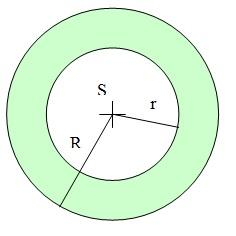Did you find an error or inaccuracy? Feel free to write us. Thank you!#### You need to know the following knowledge to solve this word math problem:

We encourage you to watch this tutorial video on this math problem:

## Related math problems and questions:

• Annulus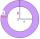Two concentric circles form an annulus of width 10 cm. The radius of the smaller circle is 20 cm. Calculate the content area of the annulus.
• Concentric circlesThere is given a Circle K with a radius r = 8 cm. How large must a radius have a smaller concentric circle that divides the circle K into two parts with the same area?
• Annulus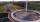Calculate the area of two circles annulus k1 (S, 3 cm) and k2 (S, 5 cm).
• Four circles1) Calculate the circle radius if its area is 400 cm square 2) Calculate the radius of the circle whose circumference is 400 cm. 3) Calculate circle circumference if its area is 400 cm square 4) Calculate the circle's area if perimeter 400 cm.
• Round skirt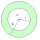The cut on the round skirt has the shape of an annulus. Determine how much m² of fabric will be consumed on an 80 cm long skirt. The circumference of the waist is a circle with a smaller radius and is 69 cm.
• Two circlesTwo circles with a radius 4 cm and 3 cm have a center distance 0.5cm. How many common points have these circles?
• Two circles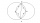Two circles with the same radius r = 1 are given. The center of the second circle lies on the circumference of the first. What is the area of a square inscribed in the intersection of given circles?
• Circle chordCalculate the length of the chord of the circle with radius r = 10 cm, length of which is equal to the distance from the center of the circle.
• Circle annulusThere are 2 concentric circles in the figure. Chord of larger circle 10 cm long is tangent to the smaller circle. What are does annulus have?Find the radius of the circle with area S = 200 cm².
• AnnulusTwo concentric circles with radii 1 and 9 surround the annular circle. This ring is inscribed with n circles that do not overlap. Determine the highest possible value of n.
• Quarter circleWhat is the radius of a circle inscribed in the quarter circle with a radius of 100 cm?Determine the radius of the circle if its perimeter and area is the same number.The radius of the larger circle is 8cm, the radius of smaller is 5cm. Calculate the contents of the annulus.Square with area 16 centimeters square are inscribed circle k1 and described circle k2. Calculate the area of circular ring, which circles k1, k2 form.XYZ is right triangle with right angle at the vertex X that has inscribed circle with a radius 5 cm. Determine area of the triangle XYZ if XZ = 14 cm.The hollow cylinder has a height of 70 cm, an outer diameter of 180 cm, and an inner diameter of 120 cm. What is the surface of the body, including the area inside the cavity?